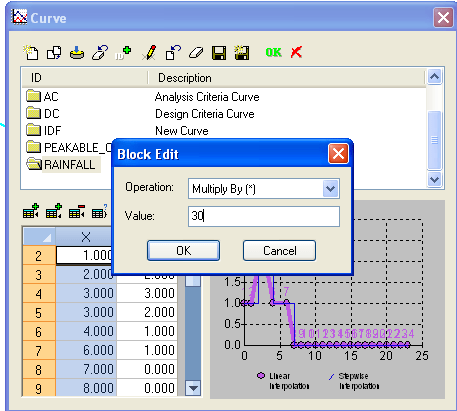InfoSewer

# RDII or Tri Triangular Unit Hydrograph in InfoSewer

Subject:  RDII or Tri Triangular Unit Hydrograph in InfoSewer

The RDII method in InfoSewer is similar to the RDII or RTK  method in  InfoSWMM with some differences.    The RTK data for triangles 1, 2 and 3 are defined in the Unit Hydrograph but instead of individual R values, the overall R is set and the Percent R1,  R2 and R3 are defined based on the total  R.  R3 is calculated internally as 100 – R1 – R2.   Each loading manhole with RDII flow has a total  area, a hyetograph and a Unit Hydrograph.  The hyetograph has to be set at multiples of the unithydrograph, so you can define the time or X columns with integers and then use the Block Edit command to change X to minutes by multiplying  by the Unit Hydrograph time (Figure 1).   You can use only one component if you set R1 or R2 to 100 percent or R3 to 100 percent by setting R1 and R2 to 0 percent (Figure 2).  The overall area of the Unit Hydrograph is divided amongst the loading manhole using the Subbasin Area (Figure 3).   The storm flows generated can be viewed using a Group Graph (Figure 4).

Figure 1.   Hyetograph Curve for the RDII Unit HydrographFigure 2.  The Unit Hydrograph is defined for various values of R, R1,  R2, T1,  T2,  T3, K1,  K2 and  K3.Figure 3.  The Unit Hydrograph and Hyetograph are tied to a particular loading manhole using a Subbasin Area.Figure 4.  The Unit Hydrographs that are generated can be viewed using a Group loading Manhole Graph.  The R1, R2 and R3 have only one triangle.

.

Categories: InfoSewer, InfoSWMM, SWMM5